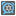• # code critique

## Question related to missionMorse Clock

Hey everyone, I finish this task with nice code. But I looking for inspection from you how to improve it.

I saw some peoples get crazy with binary that i didn't understand so much /=

```L =  (8, 4, 2, 1)
def time_maker(var,what_is_it = 4):
t = ""
T = L[-(what_is_it):]
for VAR in T:
if VAR<=var:
t+="-"
var-=VAR
else: t+="."
return t

def checkio(data):
data = [(x[:1], x[1:]) for x in data.split(':') ]
T = []
was_first = False
for [a,b] in data:
if b=="": b, a = a, '0'
what_is_it = 3
if not was_first :what_is_it, was_first = 2, True
T.append(time_maker(int(a),what_is_it)+ " "+ time_maker(int(b)))

return " : ".join(T)
```

Anyway, Thanks for the tips :-)